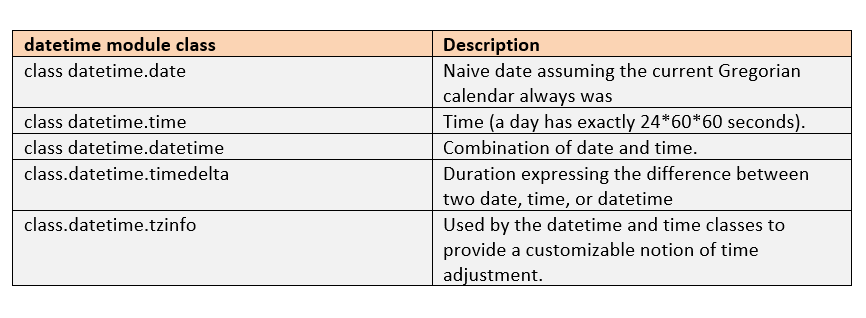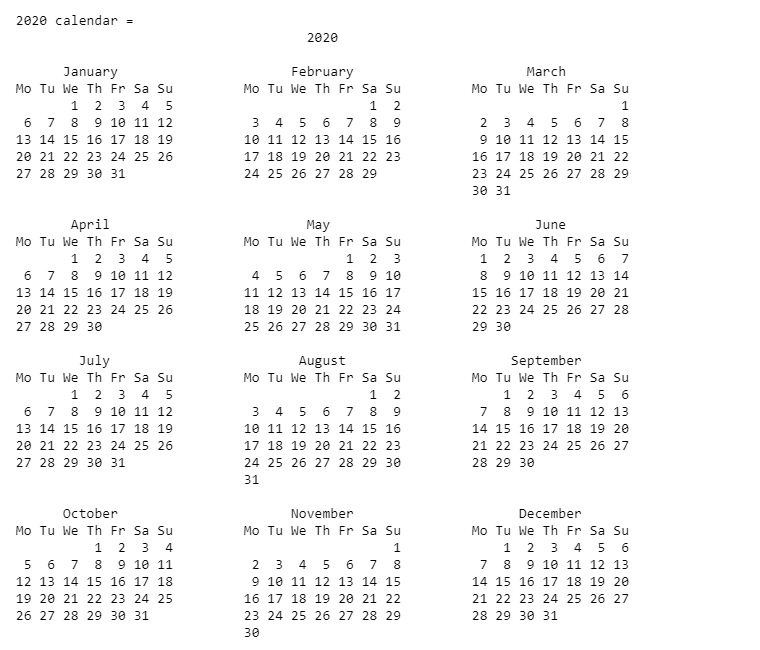## 22 Jul Python DateTime Tutorial with examples

To work with Dates in Python, import a module datetime. Let us see what datetime module is and how we can use it to manipulate date and time. Additionally, we will also work around date and time modules and understand the concept of Python DateTime.

## Python datatime module

The datetime module has classes for date and time manipulation, such as getting the current date, fetching month from date, how to fetch week, month number, etc.

The classes available in datetime module are:## Get the current date and time

To get the current date and time in Python, import the datetime module:

After that, use the now() built-in function:

Now, let us see an example and get the current date and time:

The output is as follows:

## Get Today’s Date

To get today’s Date, at first, import date class from datetime module:

Let us now see an example to get today’s date in Python:

The output is as follows:

## Get the weekday (short) in Python

To get the weekday (short) like Mon, Tue, Wed, etc. in Python, use the %a format code. Let us see an example to get the weekday:

The output is as follows:

## Get the weekday (complete) in Python

To get the weekday like Mon, Tue, Wed, etc. in Python, use the %A format code. Let us see an example to get the weekday:

The output is as follows:

## Get the weekday as a number in Python

To get the weekday as a number, such as 0 for Sunday, 1 for Monday, 2 for Tuesday. 3 for Wednesday, etc., use the %w format code. Now, let us see an example:

The output is as follows:

## Get the day of month in Python

To get the day of month, i.e. from 01 to 31, use the %d format code in Python. Now, let us see an example:

The output is as follows:

## Get the month name (short) in Python

To get the month name in short, such as Jan, Feb, Mar, etc., use the %b format code. Now, let us see an example:

The output is as follows:

## Get the month name (complete) in Python

To get the month name, such as January, February, March, April, etc., use the %B format code. Now, let us see an example:

The output is as follows:

## Display the month as a number in Python

To display the month as a number, such as 01 for January, 02 for February, 02 for March, etc., use the %m format code. Let us now see an example:

The output is as follows:

## Display year (short) in Python

To get the year (without century), such as 19 for 2019, 20 for 2020, etc., use the %y format code. Now, let us see an example:

The output is as follows:

## Display year (complete) in Python

To get the year (with century), such as 2018, 2019, 2020, etc., use the %Y format code. Now, let us see an example:

The output is as follows:

## Display hour in 24-hour format with Python

To display the hour in 24-hour format (00 – 24), such as for 18 for 6PM, 22 for 10PM, 23 for 11PM, etc., use the %H format code. Let us now see an example:

The output is as follows:

## Display hour in 12-hour format with Python

To display the hour in 12-hour format (00 – 12), such as for 6 for 6PM, 10 for 10PM, 11 for 11PM, etc., use the %I format code. However, the 24-hour format supports 0-24 format i.e. 18 for 6PM, 22 for 10PM, etc.

Let us see an example:

The output is as follows:

## Display whether the date is AM/PM in Python

To display whether the date is AM/PM, use the %p format codes in Python. Let us see an example:

The output is as follows:

## Display Day number (001-366) of year in Python

To display day number of year in Python, use the %j format code. Let us see an example:

The output is as follows:

## Get the week number of year in Python

To get the week number of year in Python, use the %U format code. Let us see an example:

The output is as follows:

## Python time module

For time related manipulation, use the time module in Python. Import the time module and use its method for manipulation:

## Get the current time

To get the current time in Python, at first, import the time module:

Next, use time() to get the current time as in the below example:

The output is as follows:

## Get the number of seconds passed since epoch

To get the number of seconds since epoch, at first, import the time module:

Note: January 1, 1970, 00:00:00 at UTC is epoch

Next, use the time() method as in the below example:

The output is as follows:

## Python calendar module

The calendar module in Python is used to perform calendar operations such as displaying calendar of a year, calendar of a month, etc.

## Display calendar of a year

To display calendar of a year, use the Calendar class. Before that, import the calendar module:

For calendar, use the calendar() method with parameters: calendar(y, width, line, col)

Moving further, let us see an example and display calendar of year 2020:

The output is as follows:## Get the calendar of a month

To get the calendar of a month in Python, import the Calendar module:

Next, let us see an example to get the calendar of let’s say, June month, year 2020:

The output is as follows:

## How to compare two dates in Python?

To compare two dates in Python, the following comparison operators are used:

Additionally, we need to use the datetime module:

Now, let us see an example to compare two dates:

The output is as follows:

In this tutorial, we learned about Python datetime, time, calendar & other modules. Additionally, we manipulated date and time with methods & format codes.

Recommended Posts[email protected]

We work to create programming tutorials for all.